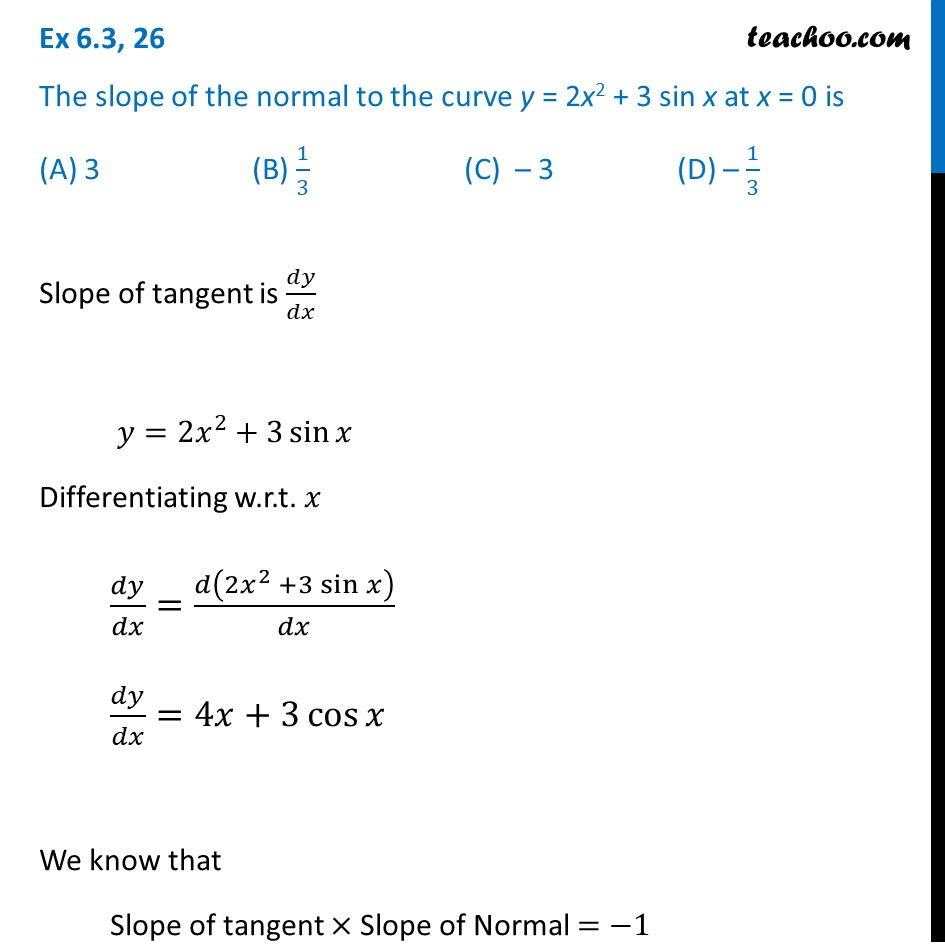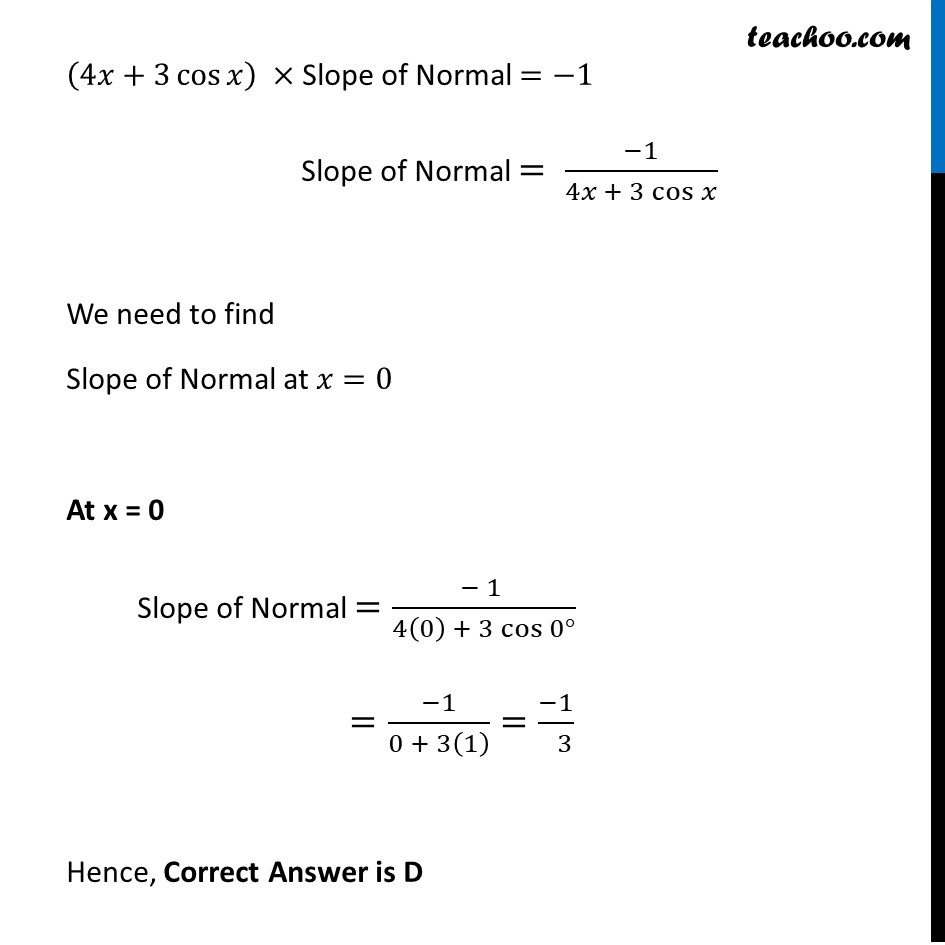Finding point when tangent is parallel/ perpendicular

Chapter 6 Class 12 Application of Derivatives
Concept wiseIntroducing your new favourite teacher - Teachoo Black, at only ₹83 per month

### Transcript

Ex 6.3, 26 The slope of the normal to the curve y = 2x2 + 3 sin x at x = 0 is (A) 3 (B) 1/3 (C) – 3 (D) – 1/3Slope of tangent is 𝑑𝑦/𝑑𝑥 𝑦=2𝑥^2+3 sin⁡𝑥 Differentiating w.r.t. 𝑥 𝑑𝑦/𝑑𝑥=𝑑(2𝑥^2 +3 sin⁡𝑥 )/𝑑𝑥 𝑑𝑦/𝑑𝑥=4𝑥+3 cos⁡𝑥 We know that Slope of tangent × Slope of Normal =−1 (4𝑥+3 cos⁡𝑥 ) × Slope of Normal =−1 Slope of Normal = (−1)/(4𝑥 + 3 cos⁡𝑥 ) We need to find Slope of Normal at 𝑥=0 At x = 0 Slope of Normal =(− 1 )/(4(0) + 3 cos⁡〖0°〗 ) =(−1)/(0 + 3(1) )=(−1)/( 3) Hence, Correct Answer is D